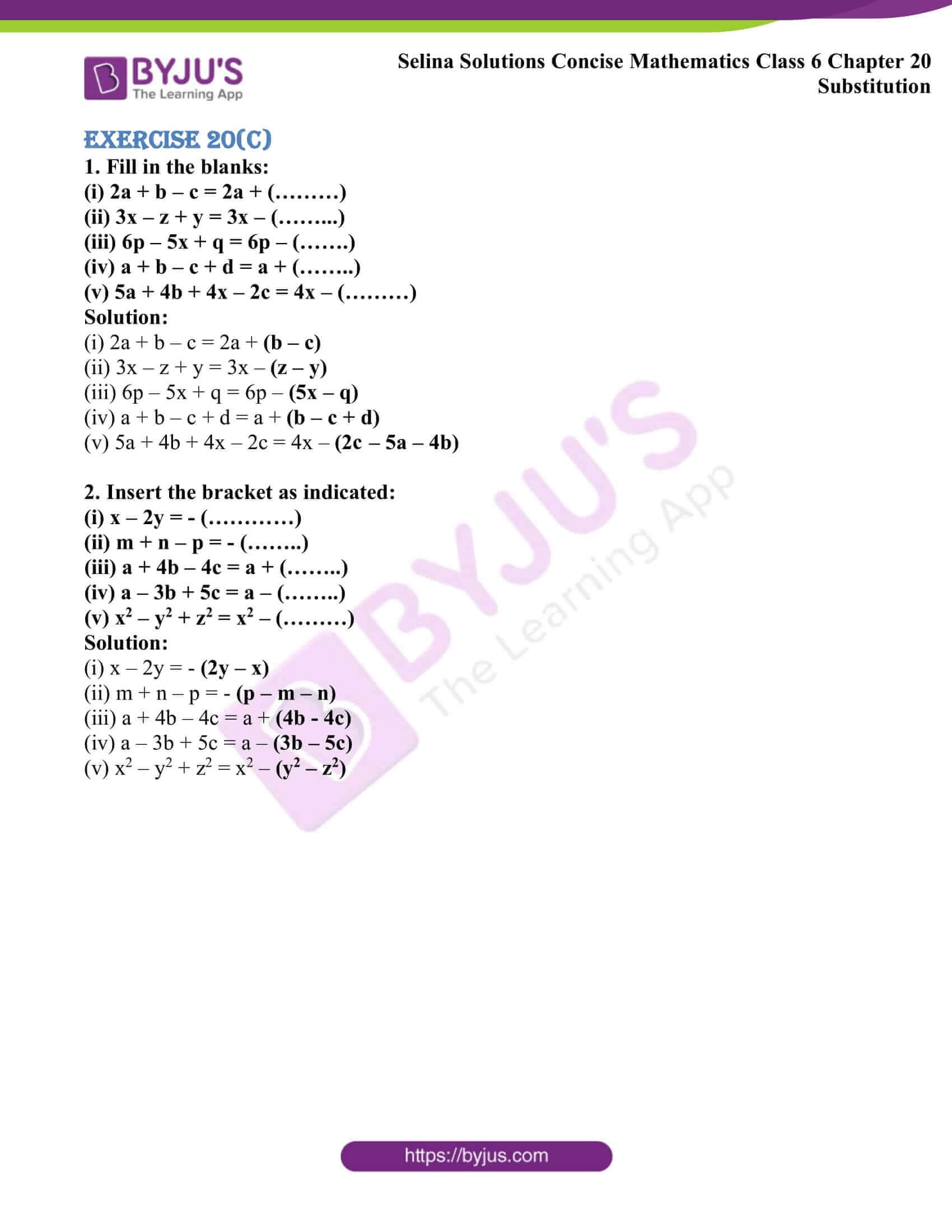# Selina Solutions Concise Mathematics Class 6 Chapter 20 Substitution Exercise 20(C)

Selina Solutions Concise Mathematics Class 6 Chapter 20 Substitution Exercise 20(C) explains the method of solving the problems by inserting the brackets. Students can make use of the opportunity to practice the problems using solutions available online and offline. The experts prepare solutions in a lucid manner, based on the current ICSE syllabus. Each step with an in-depth explanation boosts confidence and competence among students in solving complex problems with ease. In order to ace the exam, students can use Selina Solutions Concise Mathematics Class 6 Chapter 20 Substitution Exercise 20(C) PDF, from the links which are given here.

## Selina Solutions Concise Mathematics Class 6 Chapter 20 Substitution Exercise 20(C) Download PDF### Access other exercises of Selina Solutions Concise Mathematics Class 6 Chapter 20 Substitution

Exercise 20(A) Solutions

Exercise 20(B) Solutions

### Access Selina Solutions Concise Mathematics Class 6 Chapter 20 Substitution Exercise 20(C)

#### Exercise 20(C)

1. Fill in the blanks:

(i) 2a + b – c = 2a + (………)

(ii) 3x – z + y = 3x – (………)

(iii) 6p – 5x + q = 6p – (…….)

(iv) a + b – c + d = a + (……..)

(v) 5a + 4b + 4x – 2c = 4x – (………)

Solution:

(i) 2a + b – c = 2a + (b – c)

(ii) 3x – z + y = 3x – (z – y)

(iii) 6p – 5x + q = 6p – (5x – q)

(iv) a + b – c + d = a + (b – c + d)

(v) 5a + 4b + 4x – 2c = 4x – (2c – 5a – 4b)

2. Insert the bracket as indicated:

(i) x – 2y = – (…………)

(ii) m + n – p = – (……..)

(iii) a + 4b – 4c = a + (……..)

(iv) a – 3b + 5c = a – (……..)

(v) x2 – y2 + z2 = x2 – (………)

Solution:

(i) x – 2y = – (2y – x)

(ii) m + n – p = – (p – m – n)

(iii) a + 4b – 4c = a + (4b – 4c)

(iv) a – 3b + 5c = a – (3b – 5c)

(v) x2 – y2 + z2 = x2(y2 – z2)